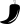# Element Search## Exercise 20 (and Solution)

Write a function that takes an ordered list of numbers (a list where the elements are in order from smallest to largest) and another number. The function decides whether or not the given number is inside the list and returns (then prints) an appropriate boolean.

Extras:

• Use binary search.

## Discussion

Topics:

1. Booleans - True and False
2. Equality testing
3. Binary search

### Booleans

When you are writing programs, there is often a time when you need to make a decision about something based on the truthfulness of something else. Basically, you need to make a decision based on whether something is TRUE or something is FALSE.

An obvious example is when using conditionals:

But what is happening behind the scenes is that the statement `michele_age > 17` is being evaluated into a type that is either `True` or `False`. This is then getting stored inside the variable `truth_value`, and then the decision is being made inside the conditional.

Here is another example:

What the `while True` statement does at the beginning of the code segment is continue asking for an age and printing a response - it never stops. (To stop it, press CTRL-C in a terminal or in the Python shell).

These types - `True` and `False` are called boolean types or boolean variables. They can only take on two values, either `True` or `False`.

For more extensive reading on Python booleans, take a look at these resources.

### Equality testing on strings

Scenarios come up where you need to test if things are equal to each other - numbers or strings or something else. We covered this idea in a previous post, but it is worth returning to again, this time for strings.

Good thing it’s easy!

Remember, comparing numbers for equality is done with an `==` sign, like so:

Turns out, comparing strings is equally (hehe..) simple, using a `==` to check for equality and `!=` to check for inequality.

Or, a more practical password-checking piece of code:

There are a number of ways to search for elements in a list, and there is no one correct way to do so. The point of this exercise is to get you thinking about possible ways to search for elements in the list, in an entire sequence of exercises about lists, searching, and sorting. This exercise might seem silly and easy at first, but the more you dive into this topic, the more difficult it becomes.

The word “binary” means there are two choices (in computers, often this is 0 or 1, but it really means any choice between two things). “Search” is to look for something. So the main idea behind binary search is to look for something in a way that gives you a decision tree for where to look, containing two choices. Let me give you an example:

Let’s take the list `a = [1, 3, 5, 30, 42, 43, 500]`. It is an “ordered list”, or a list where the elements in the list go from smaller to larger. Let’s say we want to know whether the element `9` is in the list or not. Here is what we do:

• Look at the middle element in the list - it is ‘30’. * ‘9 < 30’, so let us ignore the elements to the right of ‘30’.
• The new list we are looking at is now `[1, 3, 5]`.
• Look at the middle element in this new list - it is `3`.
• ‘9 > 3’, so ignore the elements to the left of `3`.
• The new list we are looking at is ``.
• The list has one element and it is not `9`.
• `9` is not in the list.

What the example shows is that in an ordered list, knowing how the element you are looking for compares to another element in the list splits the list in two - one half where the element can be, and one where it definitely cannot be. In the case where our list contains millions of elements, knowing that we can cut the “search space” in half is a great increase in efficiency.

When you are writing the solution, first try to write it without binary search. Then when you want to try implementing binary search, write a separate function. In the solution I will give an example for how to write a binary search in Python.

## Happy coding!

Forgot how to submit exercises?

## Share the fun!